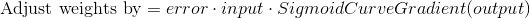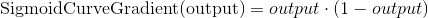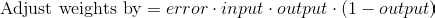Gradient descent is a first-order iterative optimization algorithm for finding the minimum of a function. To find a local minimum of a function using gradient descent, one takes steps proportional to the negative of the gradient (or of the approximate gradient) of the function at the current point.## Use of gradient descent in neural networks

In practice, gradient descent is used in neural networks during the training period for the adjustment of weights applied to input data.

It is calculated by minimizing the error between the output of the neural net (sum of input data multiplied by weights and normed with the sigmoid function) and expected output from the training data set.The sigmoid derivative equation is:So the final equation to adjust weights is:In deep neural networks, gradient descent also relies upon backpropagation, as the error needs to be calculated from the last layer of neurons to the first.

Read more on gradient descent and the adjustment of weights in neural networks in the post on programming a simple neural network or review the video on principles of neural nets.